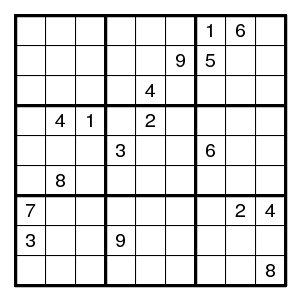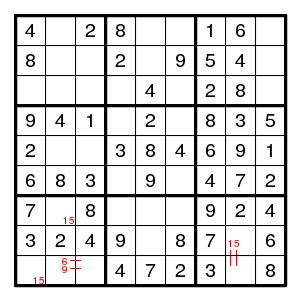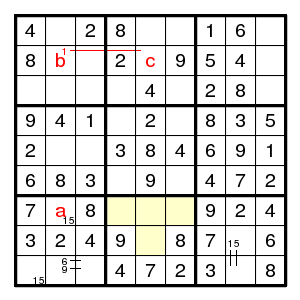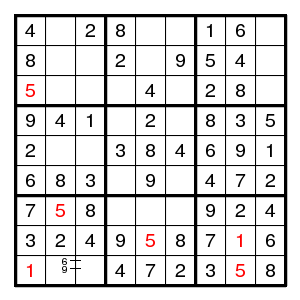Prev Up Next

# Empty rectangles

The following technique was described by Rod Hagglund. If two lines (e.g., a row and a column) cover all possible places for a given digit in some box, then that digit cannot occur on both lines outside that box, since that would not leave any place for the digit inside the box.

Terminology Rod Hagglund called the pattern involved a 'hinge'. Havard calls it an 'empty rectangle'.Starting with the given 17-clue puzzle, one easily arrives at the second diagram above.Now where should the digit 1 be in the middle bottom box? It is in one of the four yellow squares, and that means that fields a and c cannot both be 1 - that would cover all yellow squares and not leave a place for 1 in the middle bottom box. But there is a 1 in the second row, and that 1 is either in field b or in field c (because the other two empty cells in that row already see a 1 in their column). We see that if a is 1, then b is not, and c is, impossible. So, a is not 1. The rest is straightforward.

Terminology We called two positions such that at least one must contain a given digit (like b and c for the digit 1) a pair for that digit. People also use terms like 'strong pair' or 'conjugate pair'. Then two positions form a 'weak pair' if at most one contains a given digit (like a and b for the digit 1).